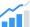# Reducing Latency, Power, and Gate Count with Floating-Point FMA

How to overcome some of the drawbacks of floating-point arithmetic units.Today’s digital signal processing applications such as radar, echo cancellation, and image processing are demanding more dynamic range and computation accuracy. Floating-point arithmetic units offer better precision, higher dynamic range, and shorter development cycles when compared with fixed-point arithmetic units. Minimizing the design’s time to market is more important than ever. Algorithm developers use MATLAB to develop and test their ideas, which are mostly floating-point arithmetic based. However, digital signal processor (DSP) programmers port the algorithms into fixed-precision arithmetic units since floating-point arithmetic units are considerably larger, slower, and more power-hungry than the fixed-point arithmetic units. This is not a trivial effort as the programmers must verify the results, including the error rate (accuracy) of fixed-point and floating-point algorithms. Furthermore, usually fixed-point software codes require more cycles than floating-point versions on many algorithms.